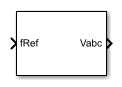# Induction Machine Scalar Control

Induction machine V/f control

•Libraries:
Simscape / Electrical / Control / Induction Machine Control

## Description

The Induction Machine Scalar Control block implements an induction machine scalar, that is V/f or V/Hz, control structure. The diagram shows the open-loop V/f control structure that the block implements.### Equations

The Induction Machine Scalar Control block computes the magnitude of the stator voltage based on the reference frequency, ${f}_{s}^{*}$, as:

`${V}_{s}^{*}=\left(\frac{{V}_{n}-{V}_{min}}{{f}_{n}-{f}_{min}}\right){f}_{s}^{*},$`

where:

• Vn is the rated voltage.

• Vmin is the minimum voltage.

• fn is the rated electrical frequency.

• fmin is the minimum frequency.

The voltage components in the stationary reference frame are:

`${V}_{\alpha }={V}_{s}^{*}\text{cos}\left(2\pi {f}_{s}^{*}t\right)$`

and

`${V}_{\beta }={V}_{s}^{*}\text{sin}\left(2\pi {f}_{s}^{*}t\right).$`

The block obtains Vabc from Vα and Vβ by using an inverse Clarke transformation.

## Ports

### Input

expand all

Reference electrical frequency.

Example: Example

Data Types: `single` | `double`

### Output

expand all

Reference phase voltages.

Example: Example

Data Types: `single` | `double`

## Parameters

expand all

Nominal frequency.

Nominal voltage.

Lower bound for the voltage.

Time, in s, between consecutive block executions. During execution, the block produces outputs and, if appropriate, updates its internal state. For more information, see What Is Sample Time? and Specify Sample Time.

If this block is inside a triggered subsystem, inherit the sample time by setting this parameter to `-1`. If this block is in a continuous variable-step model, specify the sample time explicitly using a positive scalar.

## Version History

Introduced in R2017b